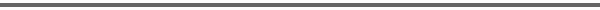Journal Home Page Cumulative Index List of all Volumes Complete Contentsof this Volume Previous Article Next Article Journal of Convex Analysis 11 (2004), No. 1, 081--094 Copyright Heldermann Verlag 2004A Local Selection Theorem for Metrically Regular Mappings A. L. Dontchev Mathematical Reviews, Ann Arbor, MI 48107-8604, U.S.A., ald@ams.org[Abstract-pdf] \def\bx{\bar x} \def\by{\bar y} \def\for{\hskip0.9pt|\hskip0.9pt} \def\lip{\mathop{\rm lip}\nolimits} \def\reg{\mathop{\rm reg}\nolimits} \def\tto{\;{\lower 1pt \hbox{$\rightarrow$}}\kern -12pt \hbox{\raise 2.8pt \hbox{$\rightarrow$}}\;} We prove the following extension of a classical theorem due to Bartle and Graves. Let a set-valued mapping $F:X \tto Y$, where $X$ and $Y$ are Banach spaces, be metrically regular at $\bx$ for $\by$ and with the property that the mapping whose graph is the restriction of the graph of the inverse $F^{-1}$ to a neighborhood of $(\by, \bx)$ is convex and closed valued. Then for any function $G:X\to Y$ with $\lip G(\bx)\cdot \reg F(\bx\for\by)) < 1$, the mapping $(F+G)^{-1}$ has a continuous local selection $x(\cdot)$ around $(\by+G(\bx),\bx)$ which is also calm. Keywords: set-valued mapping, metric regularity, continuous selection, Bartle-Graves theorem. MSC 2000: 49J53, 47H04, 54C65. FullText-pdf (450 KB) for subscribers only.